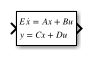# Descriptor State-Space

Model linear implicit systems

• Library:

•## Description

The Descriptor State-Space block allows you to model linear implicit systems that can be expressed in the form$E\stackrel{˙}{x}=Ax+Bu$ where E is the mass matrix of the system. When E is nonsingular and therefore invertible, the system can be written in its explicit form $\stackrel{˙}{x}={E}^{-1}Ax+{E}^{-1}Bu$ and modeled using the State-Space block.

When the mass matrix E is singular, one or more derivatives of the dependent variables of the system are not present in the equations. These variables are called algebraic variables. Differential equations that contain such algebraic variables are called differential algebraic equations. Their state space representation is of the form

`$\begin{array}{c}E\stackrel{˙}{x}=Ax+Bu\\ y=Cx+Du\end{array}$`

where the variables have the following meanings:

• x is the state vector

• u is the input vector

• y is the output vector

## Ports

### Input

expand all

Real-valued input vector of type `double` whose width is the number of columns in the B and D matrices.

Data Types: `double`

### Output

expand all

Real-valued input vector of type `double` whose width is the number of rows in the C and D matrices.

Data Types: `double`

## Parameters

expand all

Specify the mass matrix E as a real-valued n-by-n matrix, where n is the number of states in the system. E must be the same size as A. E can be singular or non-singular.

#### Programmatic Use

 Block Parameter: `E` Type: character vector, string Values: scalar | matrix Default: `'1'`

Specify the matrix coefficient A as a real-valued n-by-n matrix, where n is the number of states in the system. A must be the same size as E.

#### Programmatic Use

 Block Parameter: `A` Type: character vector, string Values: scalar | matrix Default: `'1'`

Specify the matrix coefficient B as a real-valued n-by-m matrix, where n is the number of states in the system and m is the number of inputs.

#### Programmatic Use

 Block Parameter: `B` Type: character vector, string Values: scalar | vector | matrix Default: `'1'`

Specify the matrix coefficient C as a real-valued r-by-n matrix, where n is the number of states in the system and r is the number of outputs.

#### Programmatic Use

 Block Parameter: `C` Type: character vector, string Values: scalar | vector | matrix Default: `'1'`

Specify the matrix coefficient D as a real-valued r-by-m matrix, where r is the number of outputs of the system and m is the number of inputs to the system.

#### Programmatic Use

 Block Parameter: `D` Type: character vector, string Values: scalar | vector | matrix Default: `'1'`

Specify initial condition of the block states. The minimum and maximum values are bound by the Output minimum and Output maximum block parameters.

### Tip

Simulink® software does not allow the initial condition of this block to be `inf` or `NaN`.

#### Dependencies

To enable this parameter, set the Initial condition source to `internal`.

#### Programmatic Use

 Block Parameter: `InitialCondition` Type: character vector, string Values: scalar | vector | matrix Default: `'0'`

Specify whether the output of the block has a direct dependency on the input signal. Use this parameter for systems having more than 500 continuous states in order to speed up simulation. For systems with 500 continuous states or less, Simulink automatically determines this setting.

#### Programmatic Use

 Block Parameter: `DirectFeedthrough` Type: character vector, string Values: 'True' | 'False' Default: `'True'`

Absolute tolerance for computing block states, specified as a positive, real-valued, scalar or vector. To inherit the absolute tolerance from the Configuration Parameters, specify `auto` or `-1`.

• If you enter a real scalar, then that value overrides the absolute tolerance in the Configuration Parameters dialog box for computing all block states.

• If you enter a real vector, then the dimension of that vector must match the dimension of the continuous states in the block. These values override the absolute tolerance in the Configuration Parameters dialog box.

• If you enter `auto` or –1, then Simulink uses the absolute tolerance value in the Configuration Parameters dialog box (see Solver Pane) to compute block states.

#### Programmatic Use

 Block Parameter: ` AbsoluteTolerance` Type: character vector, string Values: `'auto'` | `'-1'` | any positive real-valued scalar or vector Default: `'auto'`

Assign a unique name to each state. If this field is blank (`' '`), no name assignment occurs.

• To assign a name to a single state, enter the name between quotes, for example, `'position'`.

• To assign names to multiple states, enter a comma-delimited list surrounded by braces, for example, `{'a', 'b', 'c'}`. Each name must be unique.

• To assign state names with a variable in the MATLAB® workspace, enter the variable without quotes. A variable can be a character vector, string, cell array, or structure.

#### Limitations

• The state names apply only to the selected block.

• The number of states must divide evenly among the number of state names.

• You can specify fewer names than states, but you cannot specify more names than states.

For example, you can specify two names in a system with four states. The first name applies to the first two states and the second name to the last two states.

#### Programmatic Use

 Block Parameter: `ContinuousStateAttributes` Type: character vector, string Values: `' '` | user-defined Default: `' '`

## Block Characteristics

 Data Types `double` Direct Feedthrough `yes` Multidimensional Signals `no` Variable-Size Signals `no` Zero-Crossing Detection `no`